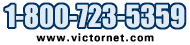Metalworking Tools and Supplies

Model # Qty

# Solving Engineering Problems in Dynamics

## Solving Engineering Problems in Dynamics

Solving Engineering Problems in Dynamics
Michael Spektor
171 Pages, Softcover
Published: April, 2014

Solving Engineering Problems in Dynamics helps practicing engineers successfully analyze real mechanical systems by presenting comprehensive methods for analyzing the motion of engineering systems and their components.
This analysis covers three basic phases:
1) composing the differential equation of motion;
2) solving the differential equation of motion; and
3) analyzing the solution.

Although a formal engineering education provides the fundamental skills for completing these phases, many engineers nonetheless would benefit by gaining further insight in using these fundamentals to solve real-life engineering problems.
This book thus describes in step-by-step order the methods related to each of these phases.

A basic education in engineering is sufficient to master the contents of this guide and effectively apply its step-by-step methods for solving engineering problems.
Numerous solutions of examples of linear, non-linear, and two-degree-of-freedom systems are found throughout.
Explains the structures of differential equations of motion of the two-degree-of-freedom systems and demonstrates the applicability of the Laplace Transform methodology for solving these equations.
Many types of engineers can benefit from this book (as well as students in mechanical, manufacturing, and industrial engineering).
Quantity Price Model Description

\$35.90 BK-SOLENG
Solving Engineering Problems in Dynamics